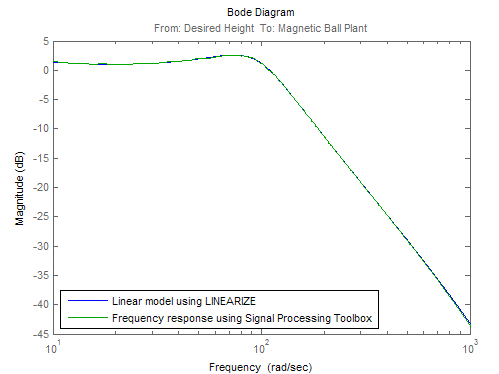## Estimate Frequency Response Models with Noise Using Signal Processing Toolbox

Open the Simulink® model, and specify which portion of the model to linearize:

```load_system('magball') io(1) = linio('magball/Desired Height',1); io(2) = linio('magball/Magnetic Ball Plant',1,'output'); ```

Create a random input signal for simulation:

`in = frest.Random('Ts',0.001,'NumSamples',1e4);`

Linearize the model at a steady-state operating point:

```op = findop('magball',operspec('magball'),... findopOptions('DisplayReport','off')); sys = linearize('magball',io,op);```

Simulate the model to obtain the output at the linearization output point:

`[sysest,simout] = frestimate('magball',io,in,op);`

Estimate a frequency response model using Signal Processing Toolbox™ software, which includes windowing and averaging:

```input = generateTimeseries(in); output = detrend(simout{1}.Data,'constant'); [Txy,F] = tfestimate(input.Data(:),... output,hanning(4000),[],4000,1/in.Ts); systfest = frd(Txy,2*pi*F); ```

Compare the results of analytical linearization and `tfestimate`:

```ax = axes; h = bodeplot(ax,sys,'b',systfest,'g',systfest.Frequency); setoptions(h,'Xlim',[10,1000],'PhaseVisible','off') legend(ax,'Linear model using LINEARIZE','Frequency response using Signal Processing Toolbox',... 'Location','SouthWest') ```In this case, the Signal Processing Toolbox command `tfestimate` gives a more accurate estimation than `frestimate` due to windowing and averaging.